# What is Machine Learning Machine learning defined Machine

• Slides: 25What is Machine Learning?Machine learning, defined: Machine learning is defined as “The process by which a computer is able to improve its own performance by continuously incorporating new data into an existing statistical model. ”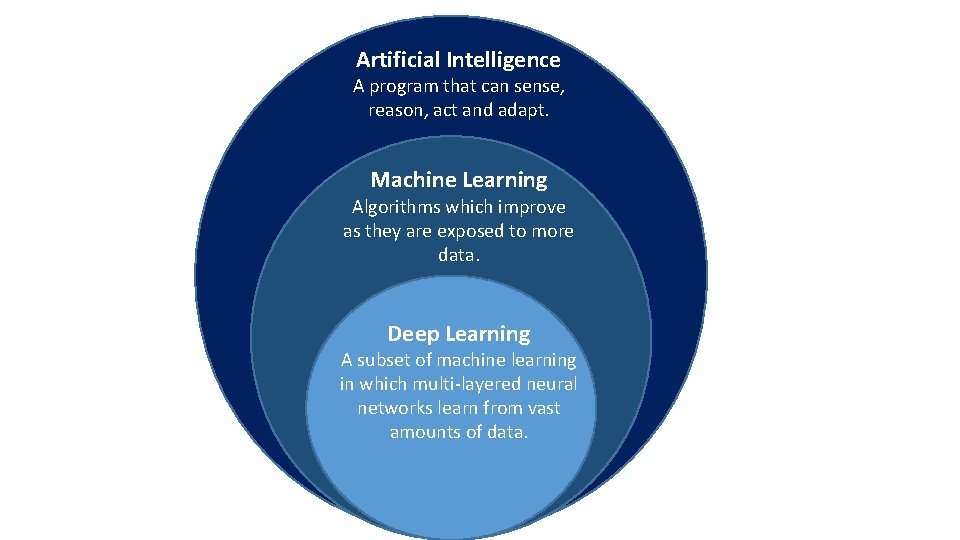Artificial Intelligence A program that can sense, reason, act and adapt. Machine Learning Algorithms which improve as they are exposed to more data. Deep Learning A subset of machine learning in which multi-layered neural networks learn from vast amounts of data.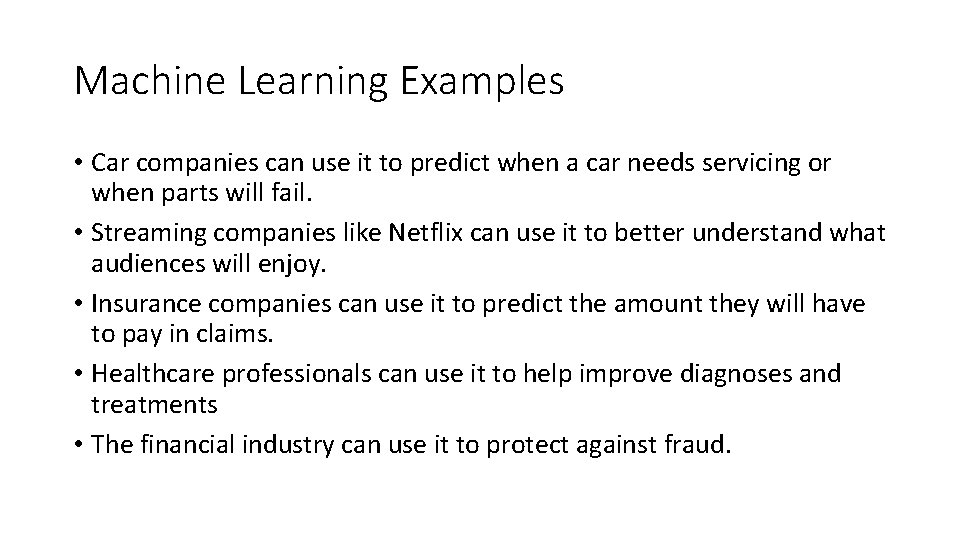Machine Learning Examples • Car companies can use it to predict when a car needs servicing or when parts will fail. • Streaming companies like Netflix can use it to better understand what audiences will enjoy. • Insurance companies can use it to predict the amount they will have to pay in claims. • Healthcare professionals can use it to help improve diagnoses and treatments • The financial industry can use it to protect against fraud.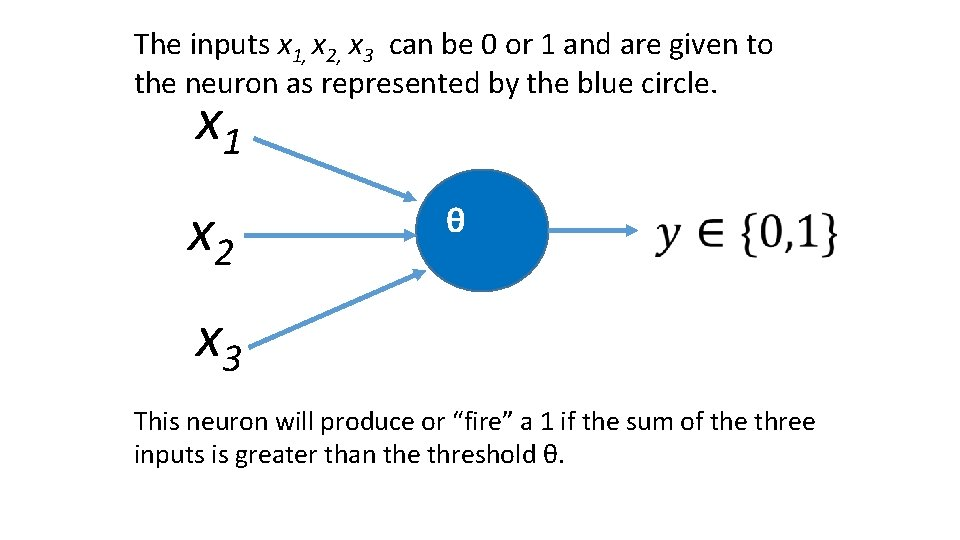The inputs x 1, x 2, x 3 can be 0 or 1 and are given to the neuron as represented by the blue circle. x 1 x 2 θ x 3 This neuron will produce or “fire” a 1 if the sum of the three inputs is greater than the threshold θ.Will I go to the football game on Friday? x 1 = Does our team have a good chance of winning? x 2 = Is the weather going to be nice? 2 x 3 = Is it a home game? The inputs x 1, x 2, x 3 can be 0 (no) or 1 (yes). This neuron will fire a 1 if the sum of the three inputs is greater than the threshold θ = 2. In other words, if any 2 of these conditions are met, I will go to the game.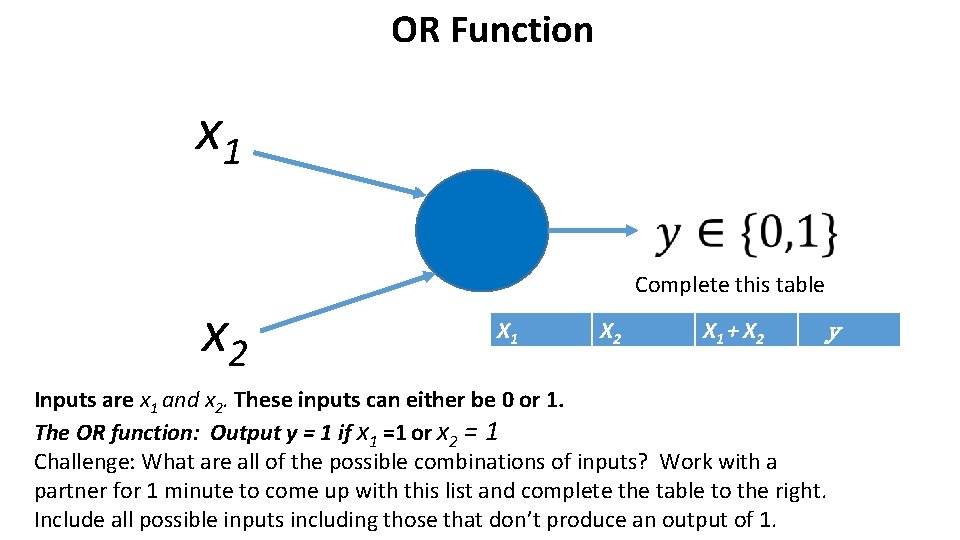OR Function x 1 x 2 Complete this table X 1 X 2 X 1 + X 2 y Inputs are x 1 and x 2. These inputs can either be 0 or 1. The OR function: Output y = 1 if x 1 =1 or x 2 = 1 Challenge: What are all of the possible combinations of inputs? Work with a partner for 1 minute to come up with this list and complete the table to the right. Include all possible inputs including those that don’t produce an output of 1.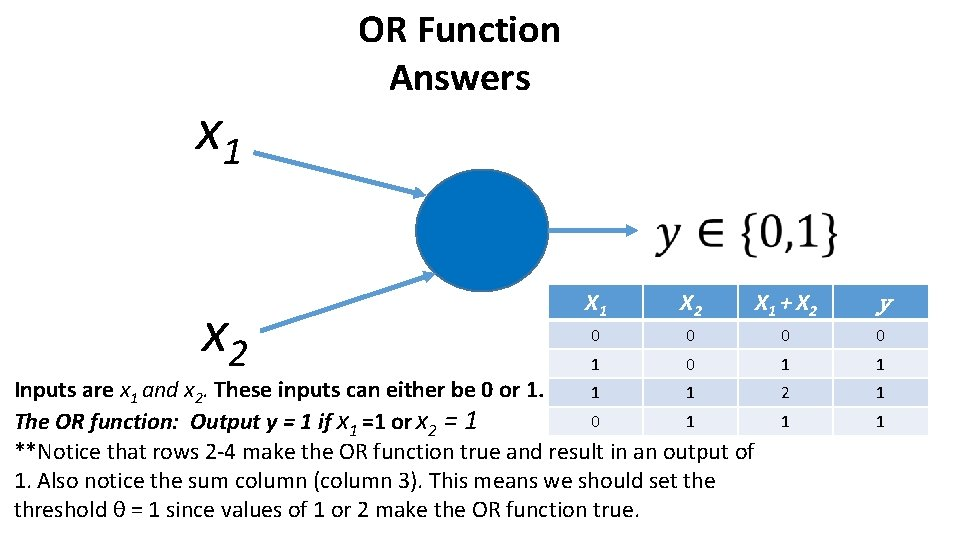x 1 OR Function Answers x 2 X 1 X 2 X 1 + X 2 y 0 0 1 0 1 1 2 1 1 1 Inputs are x 1 and x 2. These inputs can either be 0 or 1. 1 1 0 1 The OR function: Output y = 1 if x 1 =1 or x 2 = 1 **Notice that rows 2 -4 make the OR function true and result in an output of 1. Also notice the sum column (column 3). This means we should set the threshold θ = 1 since values of 1 or 2 make the OR function true.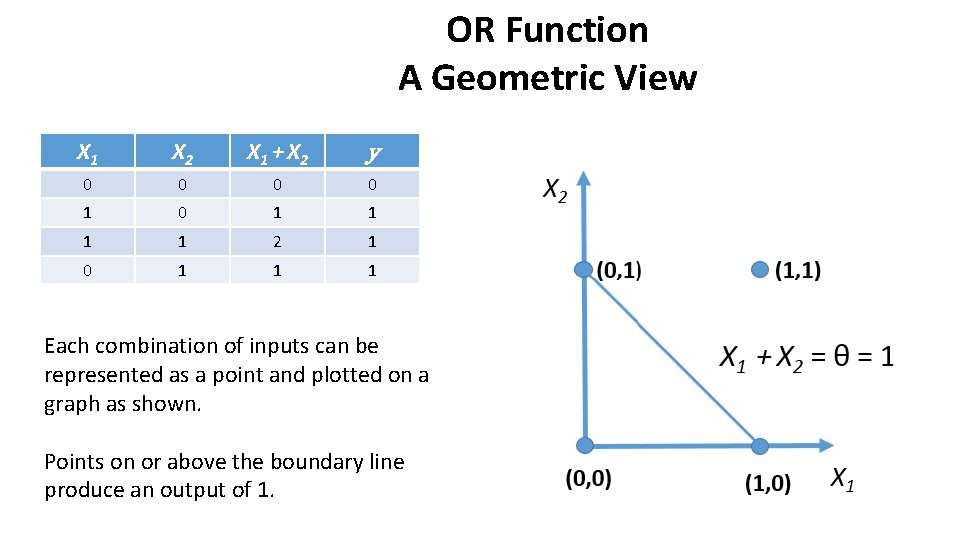OR Function A Geometric View X 1 X 2 X 1 + X 2 y 0 0 1 0 1 1 2 1 0 1 1 1 Each combination of inputs can be represented as a point and plotted on a graph as shown. Points on or above the boundary line produce an output of 1.AND Function x 1 x 2 Complete this table X 1 X 2 X 1 + X 2 y Inputs are x 1 and x 2. These inputs can either be 0 or 1. The AND function: Output y = 1 if x 1 =1 AND x 2 = 1 Challenge: What are all of the possible combinations of inputs? Work with a partner for 1 minute to come up with this list and complete the table to the right. Include all possible inputs including those that don’t produce an output of 1.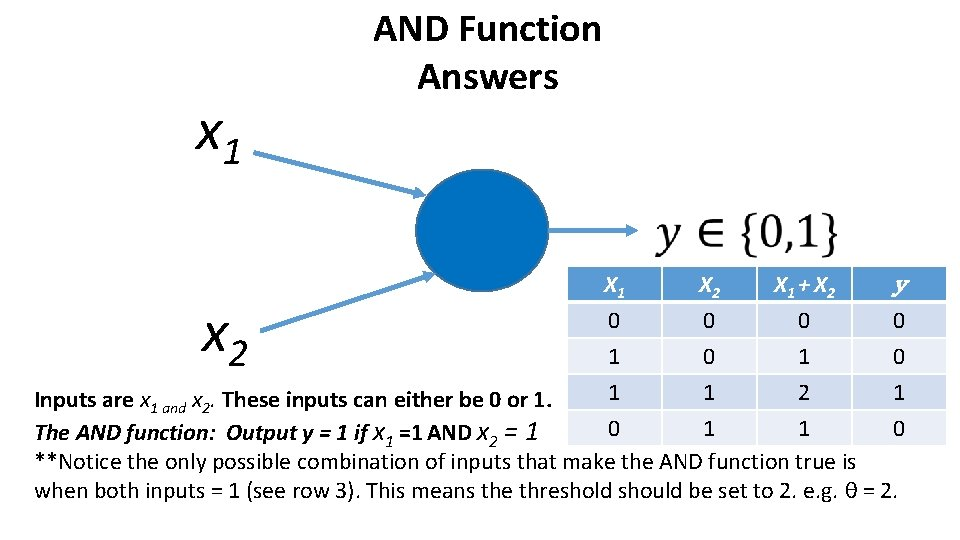x 1 AND Function Answers x 2 X 1 0 1 1 X 2 0 0 1 X 1 + X 2 0 1 2 y 0 0 1 Inputs are x 1 and x 2. These inputs can either be 0 or 1. 0 1 1 0 The AND function: Output y = 1 if x 1 =1 AND x 2 = 1 **Notice the only possible combination of inputs that make the AND function true is when both inputs = 1 (see row 3). This means the threshold should be set to 2. e. g. θ = 2.AND Function A Geometric View X 1 0 1 1 X 2 0 0 1 X 1 + X 2 0 1 2 0 0 1 1 0 y Challenge: Plot the points from the table and sketch the boundary line if it exists.AND Function A Geometric View X 1 0 1 1 X 2 0 0 1 X 1 + X 2 0 1 2 0 0 1 1 0 y The boundary line does exist, which means this is linear separable. Notice threshold is 2 in this case.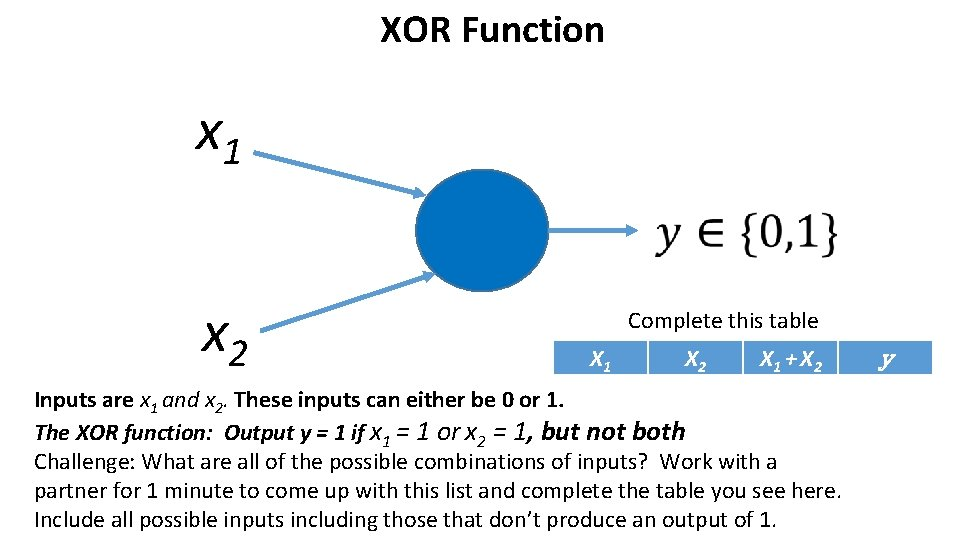XOR Function x 1 x 2 Complete this table X 1 X 2 X 1 + X 2 Inputs are x 1 and x 2. These inputs can either be 0 or 1. The XOR function: Output y = 1 if x 1 = 1 or x 2 = 1, but not both Challenge: What are all of the possible combinations of inputs? Work with a partner for 1 minute to come up with this list and complete the table you see here. Include all possible inputs including those that don’t produce an output of 1. y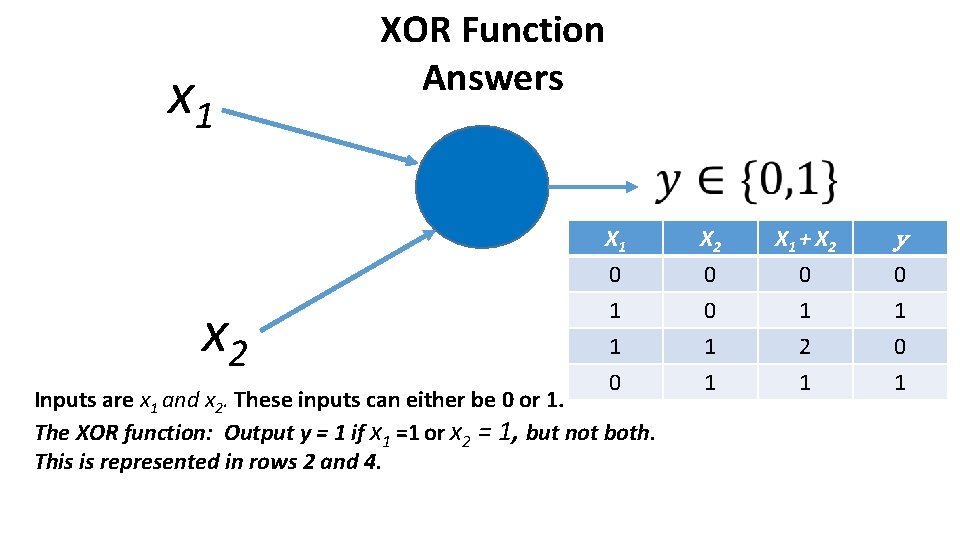x 1 XOR Function Answers x 2 X 1 0 1 1 X 2 0 0 1 X 1 + X 2 0 1 0 0 1 1 1 Inputs are x 1 and x 2. These inputs can either be 0 or 1. The XOR function: Output y = 1 if x 1 =1 or x 2 = 1, but not both. This is represented in rows 2 and 4. y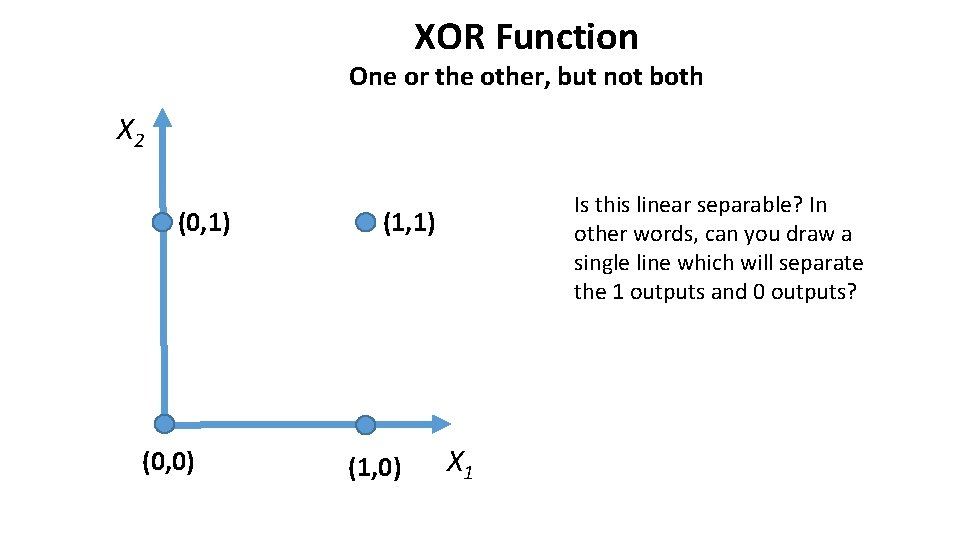XOR Function One or the other, but not both X 2 (0, 1) (0, 0) Is this linear separable? In other words, can you draw a single line which will separate the 1 outputs and 0 outputs? (1, 1) (1, 0) X 1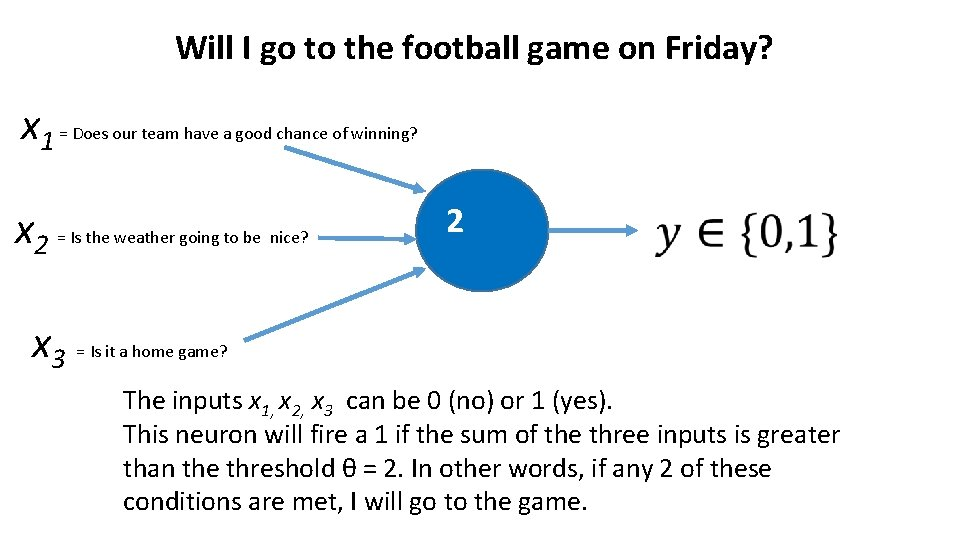Will I go to the football game on Friday? x 1 = Does our team have a good chance of winning? x 2 = Is the weather going to be nice? 2 x 3 = Is it a home game? The inputs x 1, x 2, x 3 can be 0 (no) or 1 (yes). This neuron will fire a 1 if the sum of the three inputs is greater than the threshold θ = 2. In other words, if any 2 of these conditions are met, I will go to the game.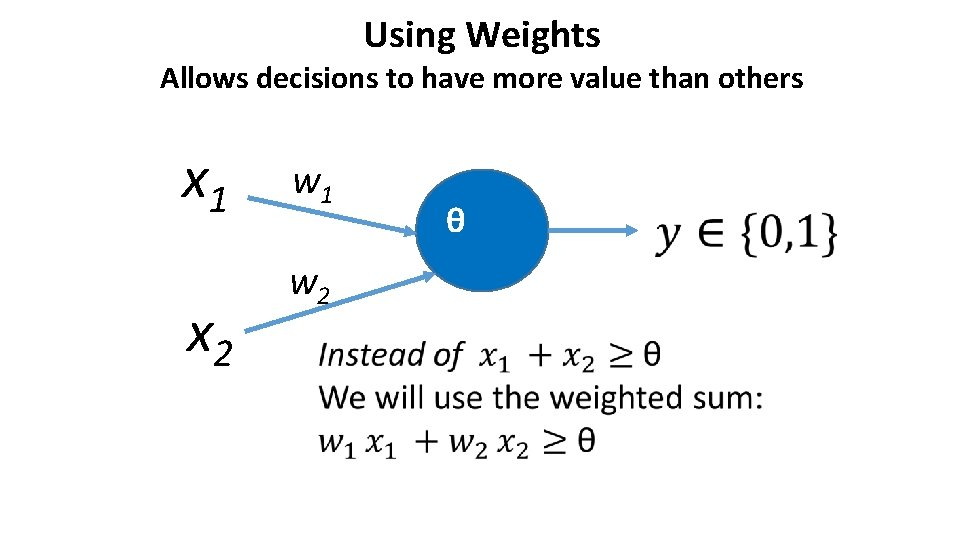Using Weights Allows decisions to have more value than others x 1 w 1 x 2 w 2 θ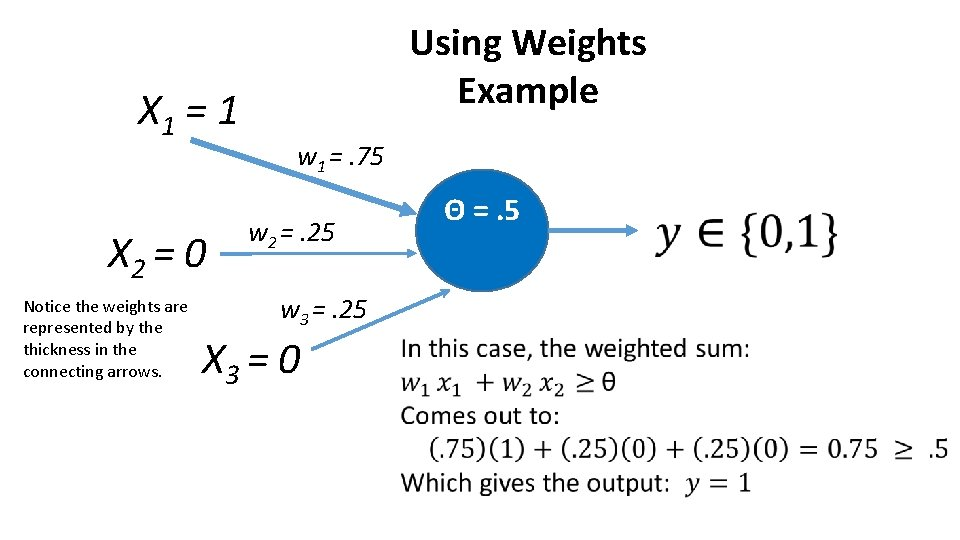X 1 = 1 X 2 = 0 Notice the weights are represented by the thickness in the connecting arrows. Using Weights Example w 1 =. 75 w 2 =. 25 w 3 =. 25 X 3 = 0 Θ =. 5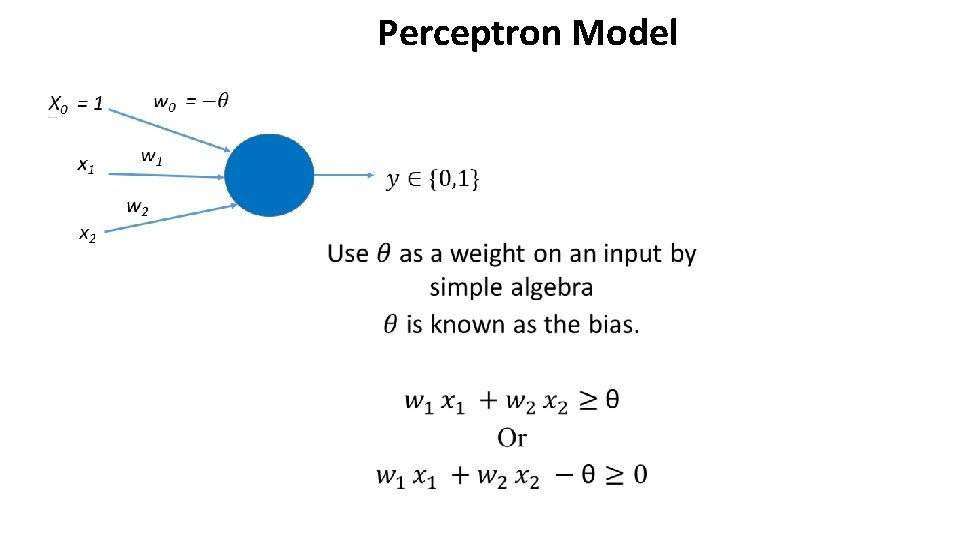Perceptron Model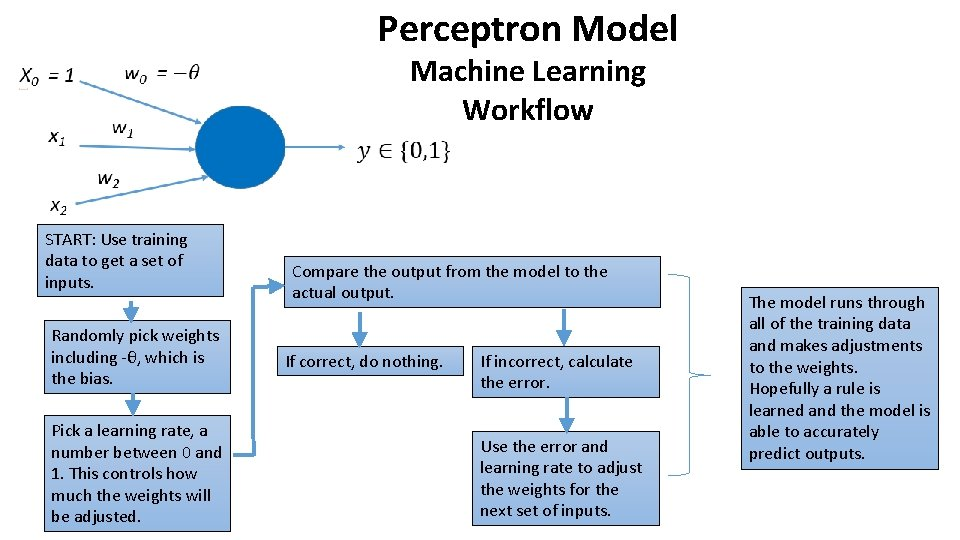Perceptron Model Machine Learning Workflow START: Use training data to get a set of inputs. Randomly pick weights including -θ, which is the bias. Pick a learning rate, a number between 0 and 1. This controls how much the weights will be adjusted. Compare the output from the model to the actual output. If correct, do nothing. If incorrect, calculate the error. Use the error and learning rate to adjust the weights for the next set of inputs. The model runs through all of the training data and makes adjustments to the weights. Hopefully a rule is learned and the model is able to accurately predict outputs.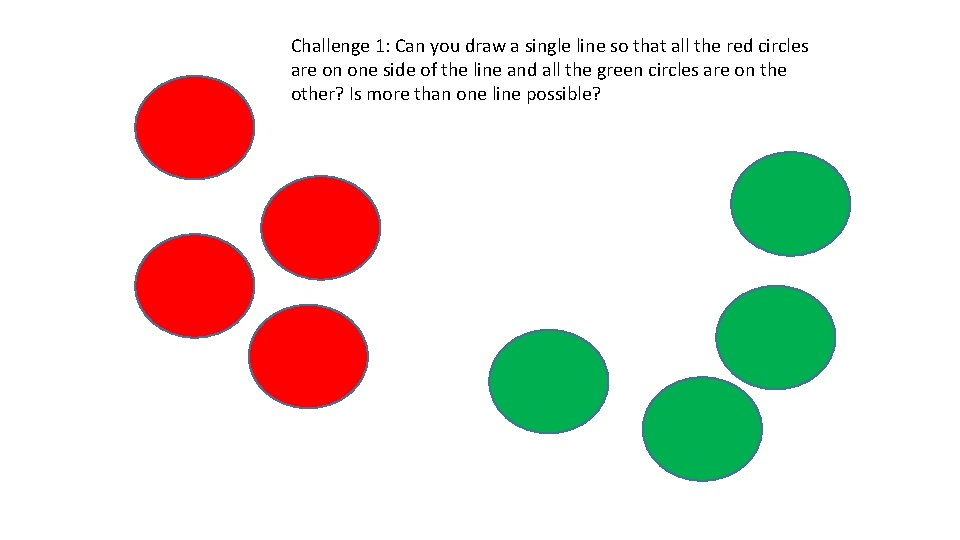Challenge 1: Can you draw a single line so that all the red circles are on one side of the line and all the green circles are on the other? Is more than one line possible?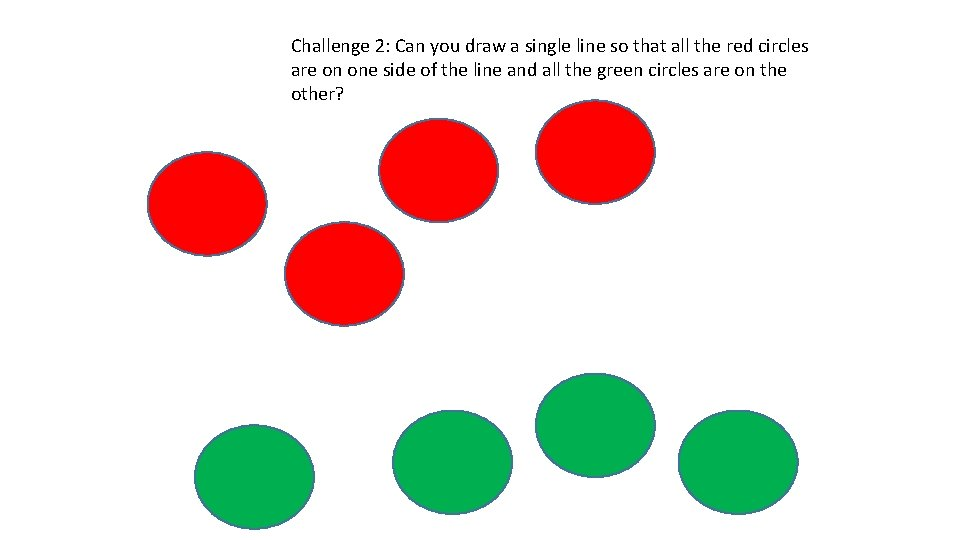Challenge 2: Can you draw a single line so that all the red circles are on one side of the line and all the green circles are on the other?Challenge 3: Can you draw a single line so that all the red circles are on one side of the line and all the green circles are on the other?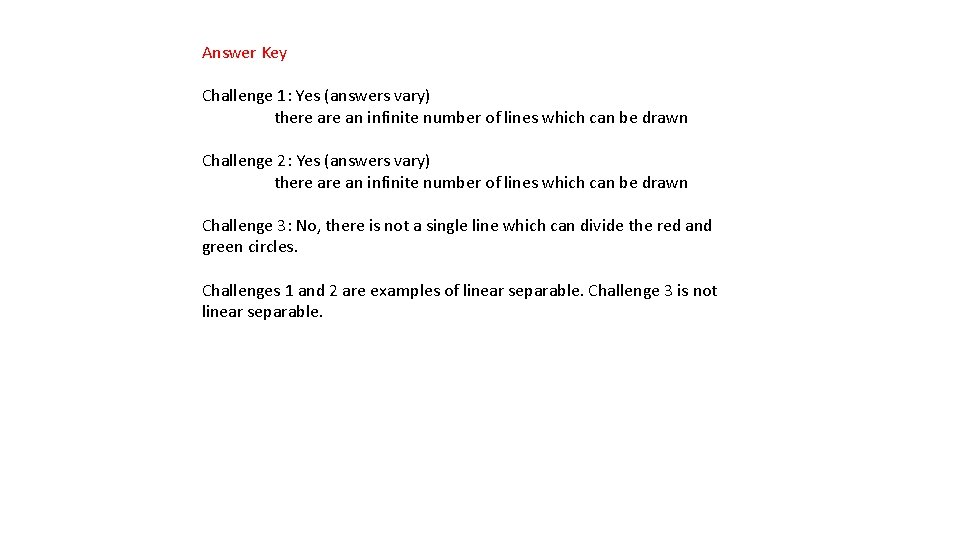Answer Key Challenge 1: Yes (answers vary) there an infinite number of lines which can be drawn Challenge 2: Yes (answers vary) there an infinite number of lines which can be drawn Challenge 3: No, there is not a single line which can divide the red and green circles. Challenges 1 and 2 are examples of linear separable. Challenge 3 is not linear separable.